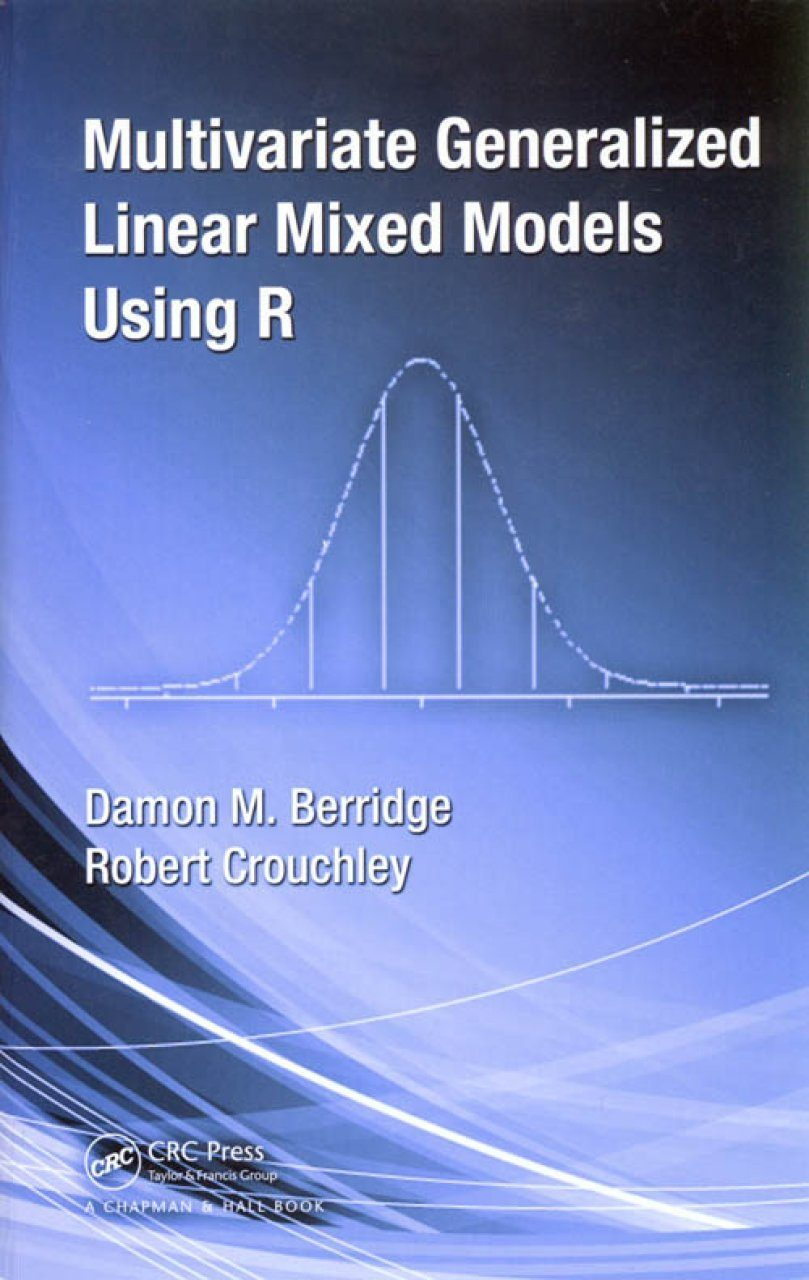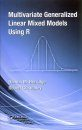## British Wildlife

6 issues per year 84 pages per issue Subscription only

British Wildlife is the leading natural history magazine in the UK, providing essential reading for both enthusiast and professional naturalists and wildlife conservationists. Published six times a year, British Wildlife bridges the gap between popular writing and scientific literature through a combination of long-form articles, regular columns and reports, book reviews and letters.

Subscriptions from £30 per year

## Conservation Land Management

4 issues per year 44 pages per issue Subscription only

Conservation Land Management (CLM) is a quarterly magazine that is widely regarded as essential reading for all who are involved in land management for nature conservation, across the British Isles. CLM includes long-form articles, events listings, publication reviews, new product information and updates, reports of conferences and letters.

Subscriptions from £18 per year

# Multivariate Generalized Linear Mixed Models Using R

By: Damon M Berridge and Robert Crouchley
280 pages, Tabs
Publisher: CRC PressClick to have a closer look
•ISBN: 9781439813263 Hardback Feb 2011 Usually dispatched within 5 days
£78.99
#188702

To provide researchers with the ability to analyze large and complex data sets using robust models, this book presents a unified framework for a broad class of models that can be applied using a dedicated R package (Sabre). The first five chapters cover the analysis of multilevel models using univariate generalized linear mixed models (GLMMs). The next few chapters extend to multivariate GLMMs and the last chapters address more specialized topics, such as parallel computing for large-scale analyses. Each chapter includes many real-world examples implemented using Sabre as well as exercises and suggestions for further reading. Appendices cover selected background topics and computing aspects.

## Contents

Introduction Generalized Linear Models for Continuous/Interval Scale Data Introduction Continuous/interval scale data Simple and multiple linear regression models Checking assumptions in linear regression models Likelihood: multiple linear regression Comparing model likelihoods Application of a multiple linear regression model Generalized Linear Models for Other Types of Data Binary data Ordinal data Count data Family of Generalized Linear Models Introduction The linear model Binary response models Poisson model Likelihood Mixed Models for Continuous/Interval Scale Data Introduction Linear mixed model The intraclass correlation coefficient Parameter estimation by maximum likelihood Regression with level-two effects Two-level random intercept models General two-level models including random intercepts Likelihood Residuals Checking assumptions in mixed models Comparing model likelihoods Application of a two-level linear model Two-level growth models Likelihood Example on linear growth models Mixed Models for Binary Data Introduction The two-level logistic model General two-level logistic models Intraclass correlation coefficient Likelihood Example on binary data Mixed Models for Ordinal Data Introduction The two-level ordered logit model Likelihood Example on mixed models for ordered data Mixed Models for Count Data Introduction The two-level Poisson model Likelihood Example on mixed models for count data Family of Two-Level Generalized Linear Models Introduction The mixed linear model Mixed binary response models Mixed Poisson model Likelihood Three-Level Generalized Linear Models Introduction Three-level random intercept models Three-level generalized linear models Linear models Binary response models Likelihood Example on three-level generalized linear models Models for Multivariate Data Introduction Multivariate two-level generalized linear model Bivariate Poisson model: Example Bivariate ordered response model: Example Bivariate linear-probit model: Example Multivariate two-level generalized linear model likelihood Models for Duration and Event History Data Introduction Duration data in discrete time Renewal data Competing risk data Stayers, Non-Susceptibles, and Endpoints Introduction Mover-stayer model Likelihood with mover-stayer model Example 1: Stayers in Poisson data Example 2: Stayers in binary data Handling Initial Conditions/State Dependence in Binary Data Introduction to key issues: heterogeneity, state dependence and non-stationarity Motivational example Random effects model Initial conditions problem Initial treatment of initial conditions problem Example: Depression data Classical conditional analysis Classical conditional model: Depression example Conditioning on initial response but allowing random effect u0j to be dependent on zj Wooldridge conditional model: Depression example Modeling the initial conditions Same random effect in the initial response and subsequent response models with a common scale parameter Joint analysis with a common random effect: Depression example Same random effect in models of the initial response and subsequent responses but with different scale parameters Joint analysis with a common random effect (different scale parameters): Depression example Different random effects in models of the initial response and subsequent responses Different random effects: Depression example Embedding the Wooldridge approach in joint models for the initial response and subsequent responses Joint model plus the Wooldridge approach: Depression example Other link functions Incidental Parameters: An Empirical Comparison of Fixed Effects and Random Effects Models Introduction Fixed effects treatment of the two-level linear model Dummy variable specification of the fixed effects model Empirical comparison of two-level fixed effects and random effects estimators Implicit fixed effects estimator Random effects models Comparing two-level fixed effects and random effects models Fixed effects treatment of the three-level linear model Appendix A: SabreR Installation, SabreR Commands, Quadrature, Estimation, Endogenous Effects Appendix B: Introduction to R for Sabre Bibliography Exercises appear at the end of most chapters.

## Biography

Damon M. Berridge is a senior lecturer in the Department of Mathematics and Statistics at Lancaster University. Dr. Berridge has nearly 20 years of experience as a statistical consultant. His research focuses on the modeling of binary and ordinal recurrent events through random effects models, with application in medical and social statistics. Robert Crouchley is a professor of applied statistics and director of the Centre for e-Science at Lancaster University. His research interests involve the development of statistical methods and software for causal inference in nonexperimental data. These methods include models for errors in variables, missing data, heterogeneity, state dependence, nonstationarity, event history data, and selection effects.
By: Damon M Berridge and Robert Crouchley
280 pages, Tabs
Publisher: CRC Press